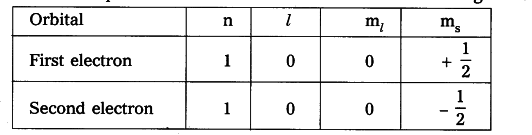# Explain Paulis exclusion principle with an example

Pauli’s exclusion principle :
No two electrons of the same atom can have all four quantum numbers the same. If n, l and m _{ l } are same for two electrons then m _{ s } must be different.
Suppose take the example of the atom.
The four quantum numbers for two electrons in the atom given below.We can observe from the table that three of the quantum numbers are same but fourth quantum number is different.So the maximum number of electrons filled in an orbital is 2.